# HOMER Pro 3.12

 Navigation: HOMER's Calculations How HOMER Calculates the Radiation Incident on the PV ArrayThe Solar GHI Resource page allows you to specify the global horizontal radiation (GHI) for each time step in the HOMER simulation. The GHI is the total amount of solar radiation striking the horizontal surface on the earth. But the power output of the PV array depends on the amount of radiation striking the surface of the PV array, which in general is not horizontal. So in each time step, HOMER must calculate the global solar radiation incident on the surface of the PV array. The following describes that process, which is based on the methods in the first two chapters of Duffie and Beckman (1991).

We can describe the orientation of the PV array using two parameters: a slope and an azimuth. The slope is the angle formed between the surface of the panel and the horizontal, so a slope of zero indicates a horizontal orientation, whereas a 90° slope indicates a vertical orientation. The azimuth is the direction towards which the surface faces. HOMER uses the convention whereby zero azimuth corresponds to due south, and positive values refer to west-facing orientations. So an azimuth of -45° corresponds to a southeast-facing orientation, and an azimuth of 90° corresponds to a west-facing orientation.

The other factors relevant to the geometry of the situation are the latitude, the time of year, and the time of day. The time of year affects the solar declination, which is the latitude at which the sun's rays are perpendicular to the earth's surface at solar noon. HOMER uses the following equation to calculate the solar declination: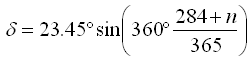where: n = the day of the year [a number 1 through 365]

The time of day affects the location of the sun in the sky, which we can describe by an hour angle. HOMER uses the convention whereby the hour angle is zero at solar noon (the time of day at which the sun is at its highest point in the sky), which is negative before solar noon, and positive after solar noon. HOMER uses the following equation to calculate the hour angle: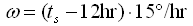where: ts = the solar time [hr]

The value of ts is 12 hr at solar noon, and 13.5 hr ninety minutes later. The equation above is based on the fact that the sun moves across the sky at 15 degrees per hour.

HOMER assumes that all time-dependent data, such as solar radiation data and electric load data, are specified not in solar time, but in civil time (also called local standard time). HOMER calculates solar time from civil time using the following equation: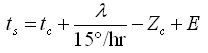where: tc = the civil time in hours corresponding to the midpoint of the time step [hr] λ = the longitude [°] Zc = the time zone in hours east of GMT [hr] E = the equation of time [hr]

Note: West longitudes are negative, and time zones west of GMT are negative as well.

The equation of time accounts for the effects of obliquity (the tilt of the earth's axis of rotation relative to the plane of the ecliptic) and the eccentricity of the earth's orbit. HOMER calculates the equation of time as follows: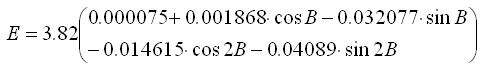where B is given by: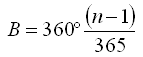where n is the day of the year, starting with 1 for January 1st.

Now, for a surface with any orientation, we can define the angle of incidence, meaning the angle between the sun's beam radiation and the normal to the surface, using the following equation: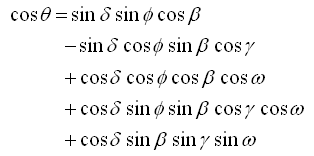where: θ = the angle of incidence [°] β = the slope of the surface [°] γ = the azimuth of the surface [°] ϕ = the latitude [°] δ = the solar declination [°] ω = the hour angle [°]

An incidence angle of particular importance, is the zenith angle, meaning the angle between a vertical line and the line to the sun. The zenith angle is zero when the sun is directly overhead, and 90° when the sun is at the horizon. Because a horizontal surface has a slope of zero, we can find a equation for the zenith angle by setting ? = 0° in the equation above, which yields: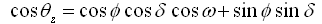where: θz = the zenith angle [°]

When addressing the amount of solar radiation arriving at the top of the atmosphere over a particular point on the earth's surface, HOMER assumes the output of the sun is constant in time. But the amount of sunlight striking the top of the earth's atmosphere varies over the year because the distance between the sun and the earth varies over the year due to the eccentricity of earth's orbit. To calculate the extraterrestrial normal radiation, defined as the amount of solar radiation striking a surface normal (perpendicular) to the sun's rays at the top of the earth's atmosphere, HOMER uses the following equation: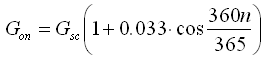where: Gon = the extraterrestrial normal radiation [kW/m2] Gsc = the solar constant [1.367 kW/m2] n = the day of the year [a number between 1 and 365]

To calculate the extraterrestrial horizontal radiation, defined as the amount of solar radiation striking a horizontal surface at the top of the atmosphere, HOMER uses the following equation: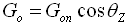where: Go = the extraterrestrial horizontal radiation [kW/m2] Gon = the extraterrestrial normal radiation [kW/m2] θz = the zenith angle [°]

Because HOMER simulates on a time-step-by-time-step basis, we integrate the equation above over one time step to find the average extraterrestrial horizontal radiation over the time step: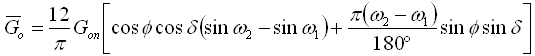where: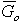= the extraterrestrial horizontal radiation averaged over the time step [kW/m2] Gon = the extraterrestrial normal radiation [kW/m2] ω1 = the hour angle at the beginning of the time step [°] ω2 = the hour angle at the end of the time step [°]

The equation above gives the average amount of solar radiation striking a horizontal surface at the top of the atmosphere in any time step. The solar resource data give the average amount of solar radiation striking a horizontal surface at the bottom of the atmosphere (the surface of the earth) in every time step. The ratio of the surface radiation to the extraterrestrial radiation is called the clearness index. The following equation defines the clearness index: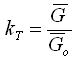where: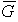= the global horizontal radiation on the earth's surface averaged over the time step [kW/m2]= the extraterrestrial horizontal radiation averaged over the time step [kW/m2]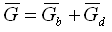where: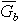= the beam radiation [kW/m2]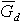= the diffuse radiation [kW/m2]

The distinction between beam and diffuse radiation is important when calculating the amount of radiation incident on an inclined surface. The orientation of the surface has a stronger effect on the beam radiation, which comes from only one part of the sky, than it does on the diffuse radiation, which comes from all parts of the sky.

However, in most cases, we measure only the global horizontal radiation, not its beam and diffuse components. For that reason, you need to enter global horizontal radiation in HOMER's Solar Resource Inputs. Then, in every time step, HOMER must resolve the global horizontal radiation into its beam and diffuse components to find the radiation incident on the PV array. For this purpose, HOMER uses correlation of Erbs et al. (1982), which gives the diffuse fraction as a function of the clearness index as follows: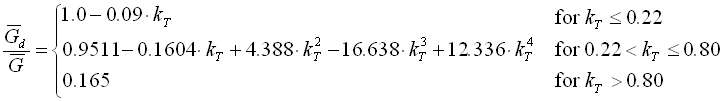For each time step, HOMER uses the average global horizontal radiation to calculate the clearness index, then the diffuse radiation. It then calculates the beam radiation by subtracting the diffuse radiation from the global horizontal radiation.

To calculate the global radiation striking the tilted surface of the PV array, HOMER uses the HDKR model, which assumes that there are three components to the diffuse solar radiation: an isotropic component that comes from all parts of the sky equally, a circumsolar component that emanates from the direction of the sun, and a horizon brightening component that emanates from the horizon. Before applying this model, we must first define three more factors.

The following equation defines Rb, the ratio of beam radiation on the tilted surface to beam radiation on the horizontal surface: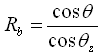The anisotropy index, with symbol Ai, is a measure of the atmospheric transmittance of beam radiation. This factor is used to estimate the amount of circumsolar diffuse radiation, also called forward scattered radiation. The anisotropy index is given by the following equation: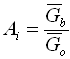Finally, we need to define a factor used to account for horizon brightening, or the fact that more diffuse radiation comes from the horizon than from the rest of the sky. This term is related to the cloudiness and is given by the following equation: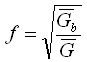The HDKR model calculates the global radiation incident on the PV array according to the following equation: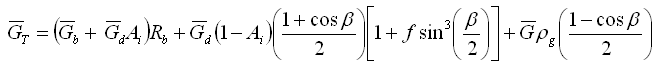where: β = the slope of the surface [°] ρg = the ground reflectance, which is also called the albedo [%]

HOMER uses this quantity to calculate the cell temperature and the power output of the PV array.